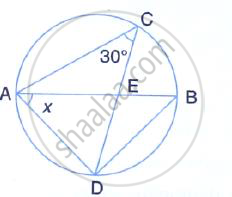Share

# In the Given Circle with Diameter Ab, Find the Value of X. - Mathematics

Course

#### Question

In the given circle with diameter AB, find the value of x.#### Solution

∠ABD = ∠ACD = 30°              [Angle in the same segment]
But, ∠ADB = 90° [ Angle in a semi-circle]
∴ x + 90° + 30° = 180°
⇒  x = 180° -120°
⇒  x = 60°

Is there an error in this question or solution?